#### Webinar: How a Strong Data and Analytics Strategy Can Help Life Sciences and Healthcare Companies Operate During the COVID-19 Crisis

Join us on June 18 at 11 am PT | 2 pm ET

# Tableau 101: Table Calculations, Part I

By: Debbie Nemirovsky | July 17, 2019

Table calculations are some of the most powerful features Tableau has to offer for answering your analytical questions. You can use a collection of pre-defined calculations or you can create your own Table Calculation from scratch using Table Calculation functions.

For this post, I wanted to find a data source that was more interesting than the usual Superstore dataset familiar to Tableau users. To create a compelling use case for this post, I started looking around online for something that appeals to my adventurous side. I visited Data.world to find this data source that holds information on all visitors to US National Parks from 1904 to 2016. As someone who spends her work days analyzing data and her spare time hiking and traveling, I was intrigued. One of my goals in life is to visit every US National Park so I wanted to answer a few questions to help me decide which parks to visit next.

Here are the questions we will answer:

• Which park is most popular? (Part I)
• Has popularity of National Parks grown over time? (Part II)
• Which park is most popular within each region? (Part II)

Note: If you graph the sum of visitors over time you will notice a spike in 2000. Any entries with no year assignment (nulls) were rolled into 2000. For a more accurate representation of the data, I suggest filtering out 2000 in any further analysis.

## Level 100 – What is a Table Calculation?

There are 3 basic types of calculations in Tableau:

• Basic expressions – These allow you to compute values at the data source level and visualization level. This means they can answer questions at granularity of the data source or at the level of detail of the visualization.
• Table calculations – These allow you to transform values at the level of detail of the visualization only.
• LOD expressions – These are similar to basic expressions but can be performed at a more granular level (INCLUDE), less granular level (EXCLUDE), or independent level (FIXED)

Here we will be focusing on how table calculations can allow us to answer questions in our visualizations.

Defining Table Calculations

Table calculations are computed locally after the results of a query are returned to Tableau desktop instead of executing in the database. These computations are applied to the returned results of a query. Since table calculations only take into consideration what you see (i.e. what is in the view) make sure to include any field needed in your calculation in your view.

The layout of your viz matters when using a table calculation. How you set up your view affects how much data you initially return from the data source and your view will determine the level of detail of your calculation.

To check if you set up your viz correctly, before using a table calculation, ask yourself these two questions:

• Do I already have all the data values I need in the visualization?
• Does the layout of the viz permit me to use a table calculation?

There are also special scenarios where only a table calculation will meet your need. These include: ranking, recursion, moving calculations, and inter-row calculations.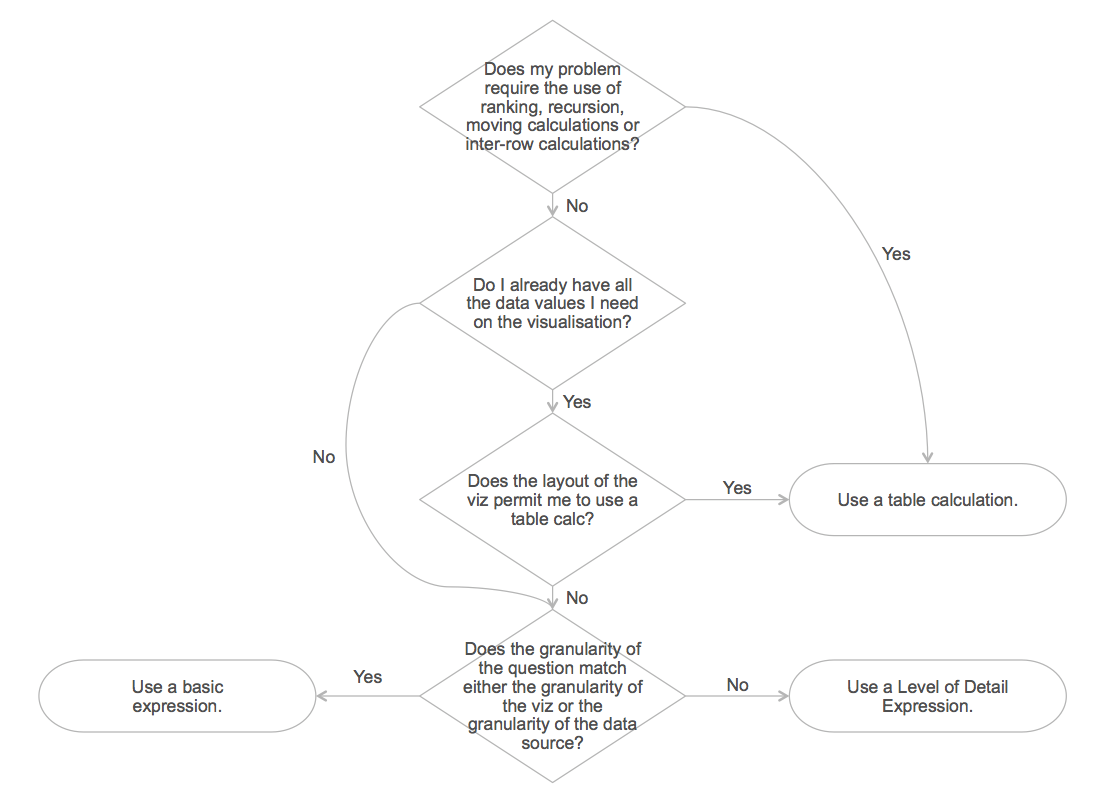### Computing the Table Calculation: Scope (Partitioning) and Direction (Addressing)

There are two critical concepts in understanding how a table calculation is executed: scope and direction, AKA partitioning and addressing. Scope is the portion of the table included in each calculation, which can be either the entire table, each pane/partition, or every individual cell in the temp table. You can think of scope as how the calculation is grouped, or how often it restarts. Direction determines the fields for which the calculation is being performed.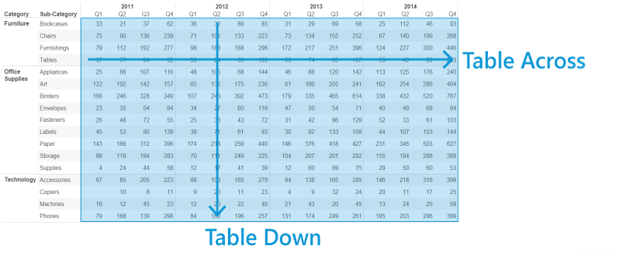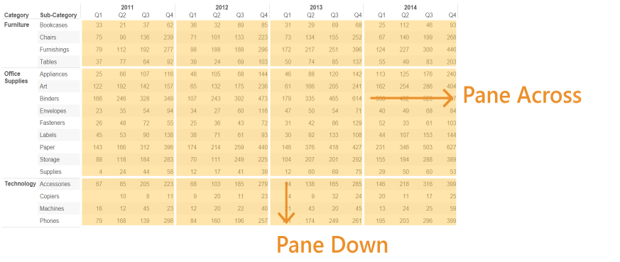Addressing and partitioning fields are the active dimension fields in the view (based on all shelves except for Filters and Tooltips shelves).

Note: You may refer to active fields as “pills.” This is a common term but not used in official Tableau documentation. We avoid this term so that you can better understand existing resources.

• Addressing fields define the direction of the calculation:
• How the calculation moves through various marks in the partition
• Defines the set of values and the sort order (for all but Rank and Total)
• Determined using the Compute Using options
• e.g. Table (Across), Table (Down), Pane (Across then Down)
• Partitioning fields define the scope of the calculation:
• Grouping for the calculation
• Tells Tableau when to start and stop the calculation and defines the buckets where a calculation will restart
• The table calculation is performed separately within each bucket/partition
• Can be the full table, a pane, a cell, a specific dimension, or customized further for an advanced calculation?
• Example: Find the average – computed across the entire view at once? Average over every year? Every month?

Note: In Tableau you define the addressing fields first. Any dimension in the view that is not an addressing field becomes part of the partitioning.

Partitioning fields group the calculation, so we would could say they represent the ‘per’ part of the expression: ‘per year’, ‘per customer’, or ‘per region and state’.

Since the addressing fields define what we are computing we could say ‘compute the percent difference for each region’, ‘compute the year-over-year growth for each national park’, or ‘compute percent of total visitors for each state’.

Now combine the phrases together to get a phrase that represents a table calculation. For example, we could say “per year, compute the percent difference for each region” or “per region, compute the year over year growth for each national park.” Later in this post I will go through an example where we define these fields.

### Using Levels of Control

There are multiple types of table calculations you can use to answer questions:

• Quick Table Calculations (Part I) – Fast and easy calculations for quickly applying a table calculation to your view. Options are simple, but limited.
• Advanced Table Calculations (Part II) – Built-in calculations with multiple options for applying a table calculation.
• Custom Table Calculations (Part II) – User-defined calculations built in the Calculated Field Editor.

We will use quick table calculations in this blog to find the most popular national park in the US.

## Level 101 – Quick Table Calculations

Quick Table Calculations are easy, “one-click” table calculations that can transform your views with little effort. Once a table calculation is applied, a delta symbol will appear to the right of the field name.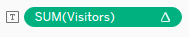Quick table calculations are a fast way of creating advanced calculations without a need to understand the underlying logic.

There are several built-in quick table calculations. Here are some examples:

• Running Total
• Difference
• Percent Difference
• Percent of Total
• YTD Growth

Now that you know the general logic of a table calculation, you can use a quick table calculation to answer questions. In this example, we are going to answer the question: Which park is most popular?

To answer this question, we will look at the number of visitors to each park over time.

### Creating a Quick Table Calculation:

First, set up your view to contain the fields you need in your calculation. Then, apply a quick table calculation.

• Create a new workbook and connect to All National Parks Visitation 1904-2016.csv
• Drag Year to columns
• Drag Region and Unit Name to Rows
• Drag Visitors to text on the marks card
• [Note: for this example, I filtered the view by Year (2012-2016) and Unit Type (National Parks and National Historical Park and Preserve), then excluded Denali National Preserve because it held the same data as Denali National Park]
• Right-click of Sum(Visitors), select Quick Table Calculation, then Rank. Compute Using Table (Down) to see the most popular national park for each year (Rank 1).

Based on this viz, we can see that the Great Smoky Mountains National Park is the most popular from 2012 – 2016.

Now that we have seen a quick table calculation in action, we will dive deeper into how these calculations work in the view. The examples below show a few different combinations of scope and direction.

### Setting up Scope and Direction of a Calculation

When using a table calculation, you can set the scope and direction of the calculation:

• Right-click Sum(Visitors), select Compute Using in dropdown menu, and then select one of the options illustrated below.

Note: Ensure Show calculation assistance is selected to show the direction of the calculation as shown below. The calculation assistant will help you visualize the effect of your selections in the Table Calculation Editor by numbering the cells or marks in the direction of the calculation, and highlighting its scope.

• Table (across)
• Computes across the length of the table (moving horizontally) and restarts each partition
• Fields that span horizontally are the addressing fields, all other fields are partitioning fields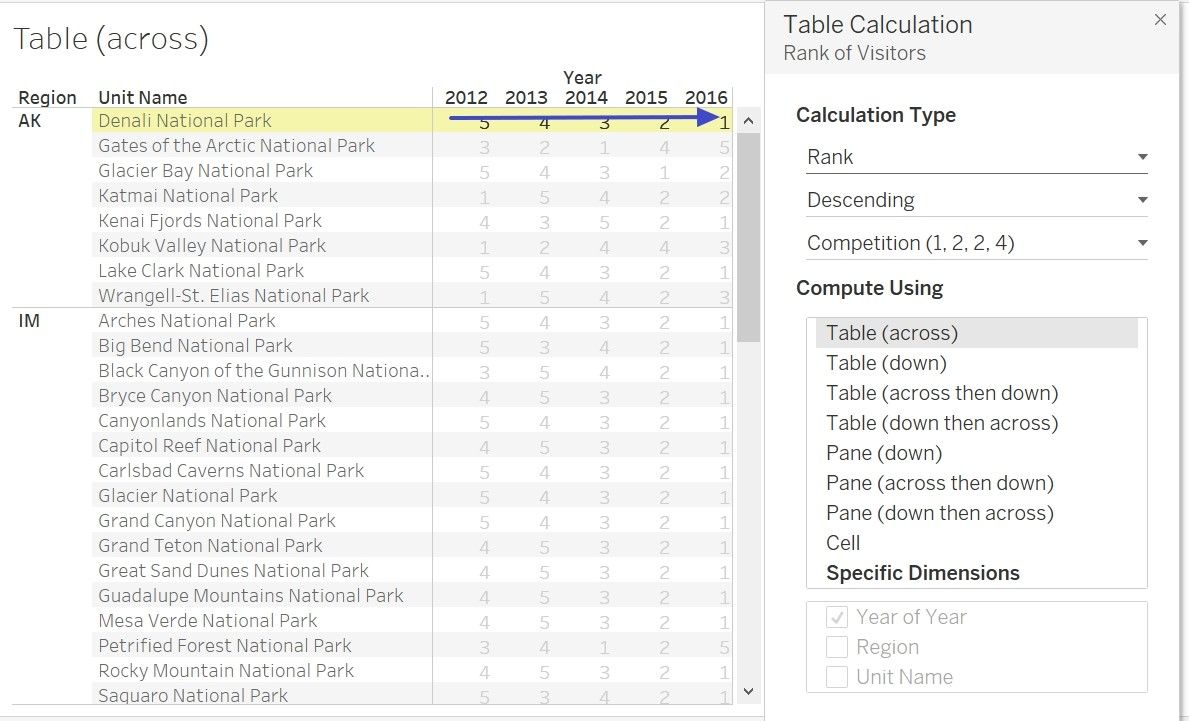• Table (down then across)
• Computes down the length of the table (moving vertically) and then across the length of the table (moving horizontally)
• The fields that span down the table and across the table are addressing fields and the entire table is the partition.
• The calculation is computed downwards, moves to the next column, computes downwards, and continues through the rest of the view.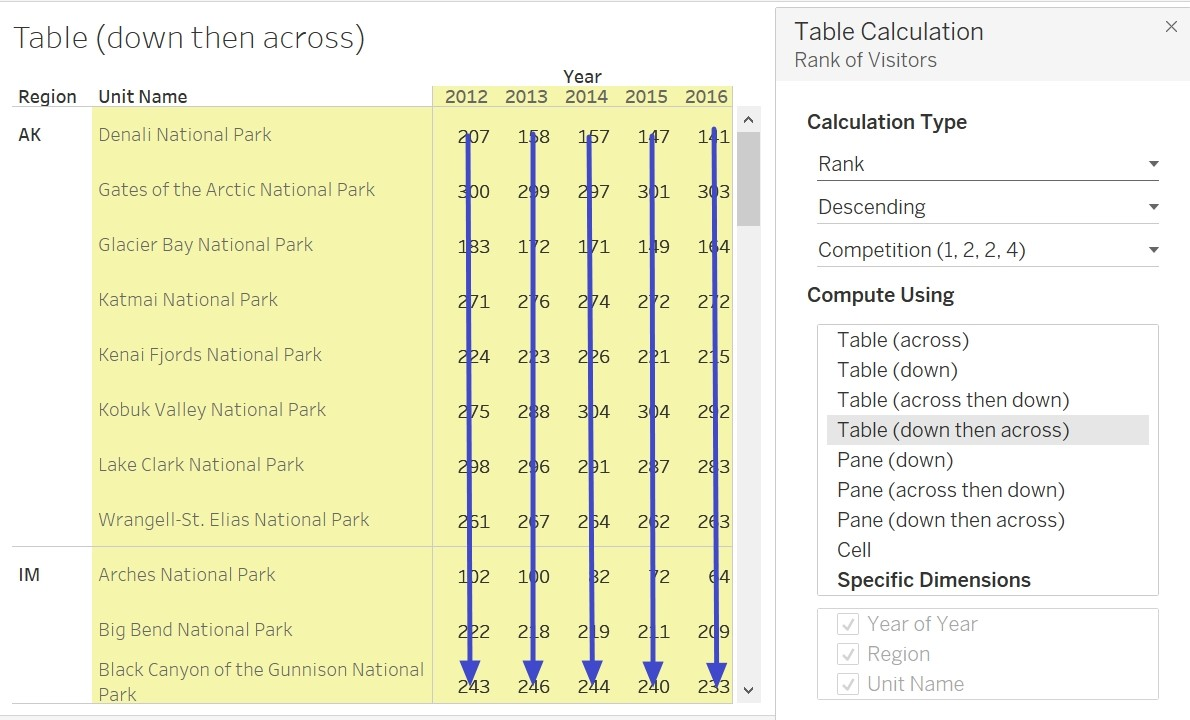• Pane (down)
• Computes down the length of the pane (moving vertically) and restarts each partition
• The fields that span down the pane are addressing fields and the fields that separate the panes are partitioning fields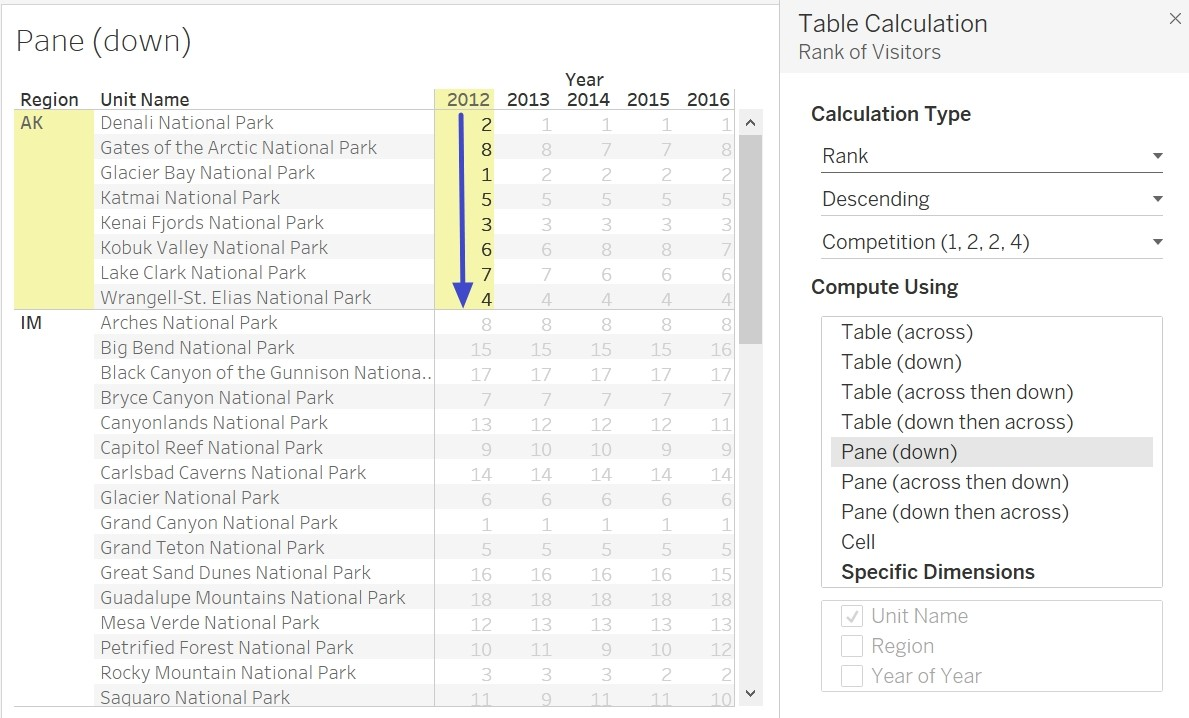Note:
If you are following along and do not get this view: 1. Go to Analysis tab, Table Layout, and select ‘Show Empty Rows.’ 2. Bring Unit Type filter to context (right-click on field in filters pane and select ‘Add to Context’)

.

• Cell
• Computes within a single cell in the view
• All fields become partitioning fields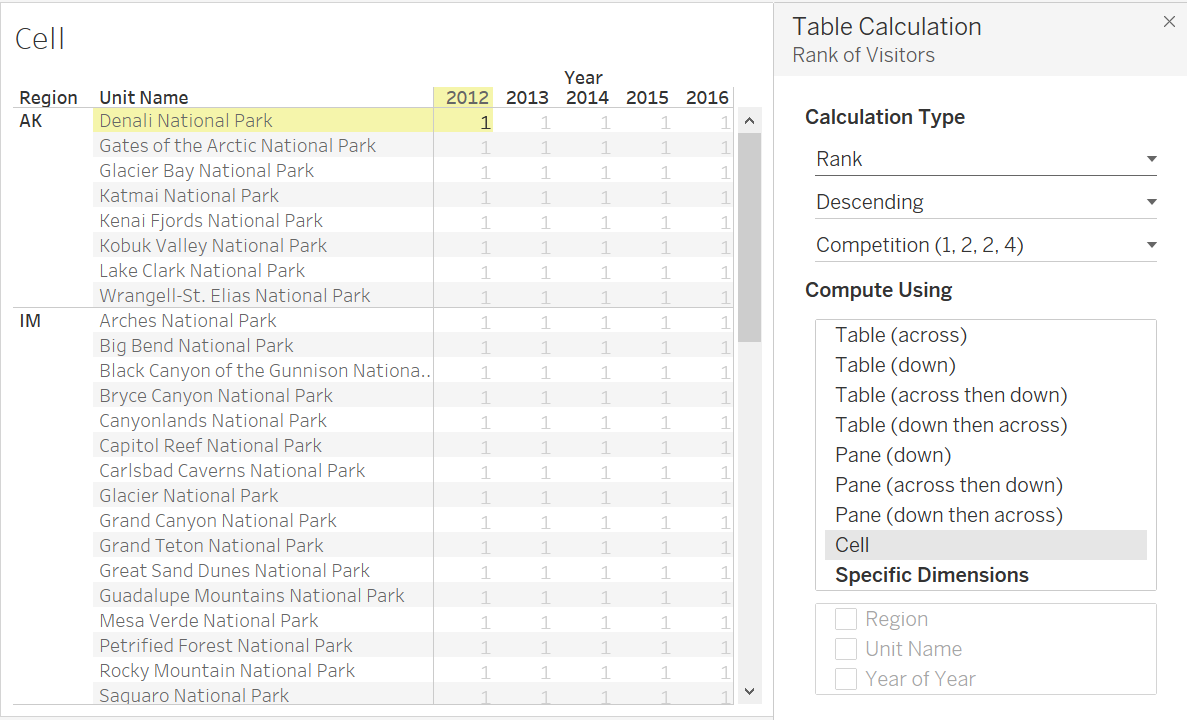• Specific Dimensions – computes only within the dimensions you specify
• Provides more flexibility than the other Compute Using options
• The order of the fields selected specifies the direction the calculation moves through various marks in the partition
• In this example the dimension checked is Year of Year which is the same direction as Table (across) since Rank is being computed across years for each Unit Name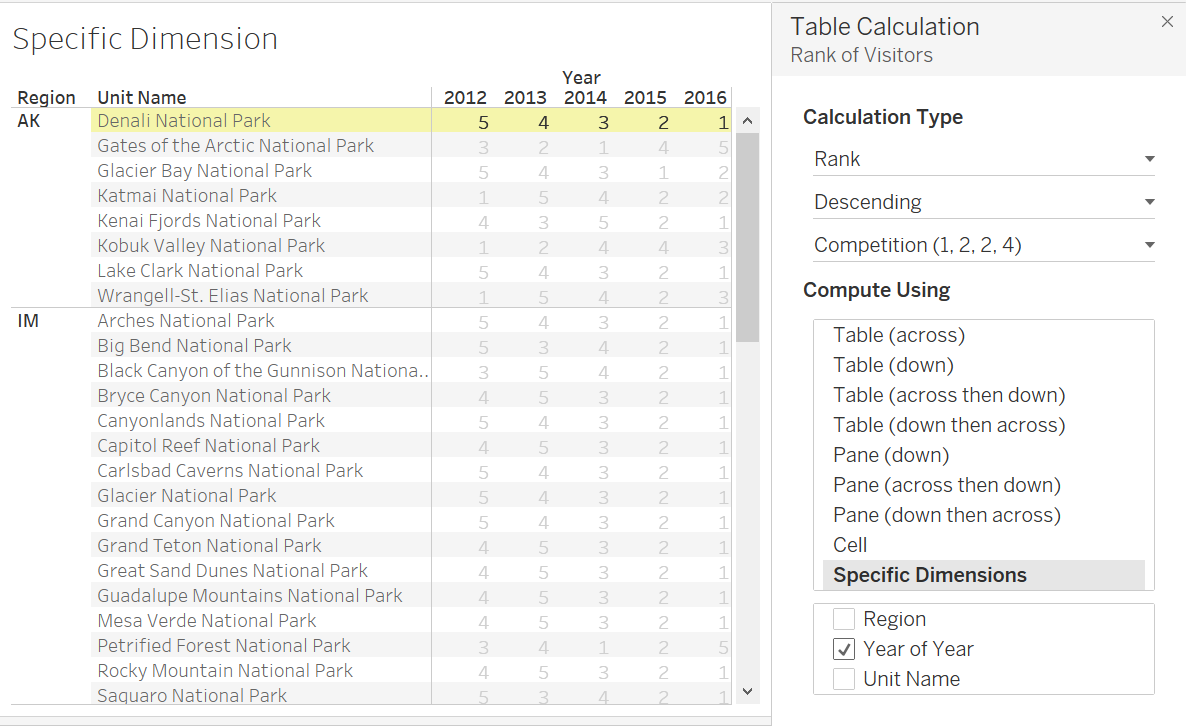When you add a table calculation with the Compute Using options, Tableau identifies dimensions as addressing and partitioning automatically, based on your selection. However, when using Specific dimensions, you are in control of choosing the addressing and partitioning fields.

Let’s circle back to our original question: Which park is most popular? Using Table (Across) Great Smoky Mountains National Park is the most popular from 2012 – 2016. You can, however, get a different answer depending on how you compute your calculation.

Your answer will vary depending on which quick table calculation computation you are using. If you’re using Table (across) then you are computing the rank of each park over time so you will find the most popular year for a particular park. If you use Table (Down) you are computing ranks for each year so you will find out which park is most popular in each year. If you are using Pane (Down) you are computing the rank of parks within each region. Here, you will find out which park is most popular within a region, for each year. To find the most popular park, across all regions, across all years use Table (Across then Down) or Table (Down then Across).

Table calculations are powerful because of this flexibility and there are endless options for these calculations to be computed.

Quick table calculations are a quick but powerful calculation that can transform your data and visualizations. We can use these calculations to answer questions in our data quickly and easily.

Stay tuned for Part II of this blog post where we will be discussing advanced and custom table calculations. We will use these powerful analytical tools to better understand the popularity and attendance at US national parks.